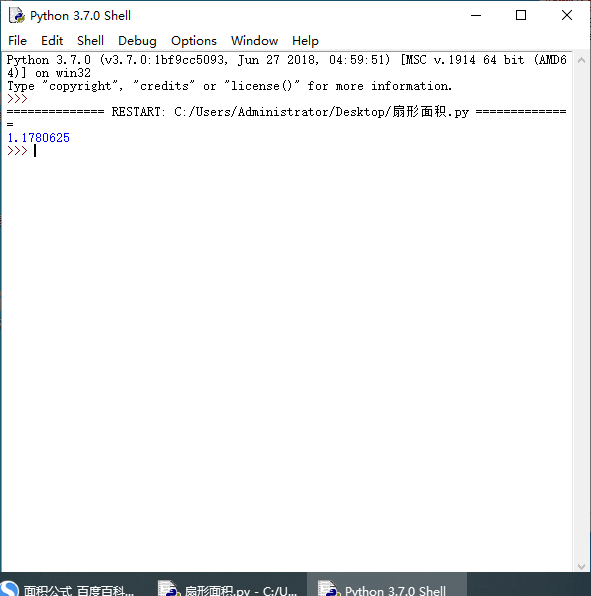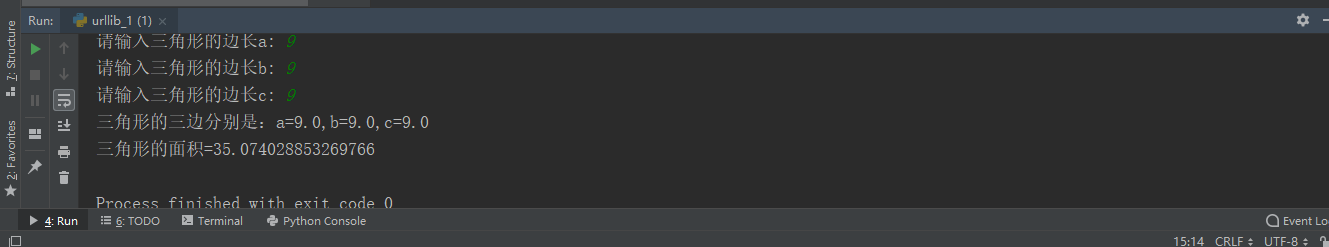• ## python求扇形面积

千次阅读 2020-03-08 09:42:57
python 扇形面积 程序如下 #sxmj.py r=1 #半径为1 area=135*3.1415*r*r/360 #135°的扇形面积 print(area) 运行结果
python 求扇形面积

程序如下

#sxmj.py
r=1 #半径为1
area=135*3.1415*r*r/360 #求135°的扇形面积
print(area)



运行结果展开全文• Python求面积周长 要求: 输入圆的半径r，计算圆的面积(area)和周(circumference)。 注意: 所有数保留两位小数。π取值3.14。方法为：print(“%.2f” % a)。 # 请使用 input() 输入圆的半径 r pi = 3.14 r = float...
• ## python求三角形面积

万次阅读 2018-11-02 10:59:52
Python第一课 目前正在自学python,上来做点笔记。...题目：已知三条边，如何一个三角形的面积? 在这里插入代码片 import math a=4.0 b=3.0 c=5.0 d=(a+b+c)/2 s=math.sqrt(d*(d-a)*(d-b)*(d-c)) print (s) ...
Python第一课
目前正在自学python,上来做点笔记。
题目：已知三条边，如何求一个三角形的面积?
代码如下：
import math
a=4.0
b=3.0
c=5.0
d=(a+b+c)/2
s=math.sqrt(d*(d-a)*(d-b)*(d-c))
print (s)

改进一下：
import math
a=float(input("请输入三角形的边长a: "))
b=float(input("请输入三角形的边长b: "))
c=float(input("请输入三角形的边长c: "))
d=(a+b+c)/2
area=math.sqrt(d*(d-a)*(d-b)*(d-c));
print(str.format("三角形的三边分别是：a={0},b={1},c={2}",a,b,c))
print(str.format("三角形的面积={0}",area))

测试结果如下：展开全文• import math a = int(input(“a=”)) b = int(input(“b=”)) c = int(input(“c=”)) flag = False if a+b>c and a+c>b and b+c>a and a>0 and b>...print(f’三角形面积为：{S}’) else: p
import math
a = int(input(“a=”))
b = int(input(“b=”))
c = int(input(“c=”))
flag = False
if a+b>c and a+c>b and b+c>a and a>0 and b>0 and c>0:
flag = True
if flag:
p = (a+b+c)/2
S = math.sqrt(p*(p-a)(p-b)(p-c))
print(f’三角形面积为：{S}’)
else:
print(‘不构成三角形’)


展开全文• 【问题描述】 ...计算由海岸线围起来的小岛面积（即：由1围起来的区域中0的个数）。如下图所示6X6方阵表示的小岛面积为9： 0 0 0 1 0 0 0 0 1 0 1 0 0 1 0 0...


【问题描述】

用一个二维方阵（最小为3X3，最大为9X9）表示一片海域。方阵中的元素只由0和1组成。1表示海岸线。计算由海岸线围起来的小岛面积（即：由1围起来的区域中0的个数）。如下图所示6X6方阵表示的小岛面积为9：
0 0 0 1 0 0
0 0 1 0 1 0
0 1 0 0 0 1
1 0 0 0 1 0
1 0 1 0 1 0
1 1 0 1 1 1
上述方阵表示的海域满足下面两个要求：
1、小岛只有一个。
2、用1表示的海岸线肯定可以封闭成一个小岛，但有可能是凸的，也有可能是凹的。所以在判断时：对于方阵中的任意一个元素0，如果其位于同一行上的两个1之间，并且位于同一列上的两个1之间，则该元素肯定在1围起来的区域中。不符合该规定的其它情况不考虑。

【输入形式】

先从标准输入中输入方阵的阶数，然后从下一行开始输入方阵的元素（只会输入0或1），各元素之间以一个空格分隔，每行最后一个元素后没有空格，但会有回车换行符。

【输出形式】

在标准输出上输出用整数表示的小岛面积。

【输入样例】

6
0 0 0 1 0 0
0 0 1 0 1 0
0 1 0 0 0 1
1 0 0 0 1 0
1 0 1 0 1 0
1 1 0 1 1 1

【输出样例】

9

【样例说明】

输入是6X6的方阵。该方阵中由1围起来的区域内有9个0，所以输出的小岛面积为9。注意：最下方的三个元素1（即第5行第3列的1、第6行第2列的1、第6行第4列的1）组成了一个凹形的海岸线，第6行第3列的0不在海岸线内，所以不应算作小岛面积。

n = int(input())
sea = []
for r in range(n):
sea.append(input().split())
# print(sea)

#求出各行的最左边1和最右边1的坐标
row_left_right = []
for r in range(n):
left_right = [None, None]
for c in range(n):
if sea[r][c] == '1':
if left_right == None:
left_right = c
if left_right == None or left_right < c:
left_right = c
row_left_right.append(left_right)
# print(row_left_right)
#求出各列的最上方1和最下端1的坐标
column_upper_lower = []
for c in range(n):
upper_lower = [None, None]
for r in range(n):
if sea[r][c] == '1':
if upper_lower == None:
upper_lower = r
if upper_lower == None or upper_lower < r:
upper_lower = r
column_upper_lower.append(upper_lower)
# print(column_upper_lower)

def is_land(row, column, row_left_right, column_upper_lower):
if row_left_right[row] == None:
return False
if column_upper_lower[column] == None:
return False
if row_left_right[row] < column < row_left_right[row] and \
column_upper_lower[column] < row < column_upper_lower[column]:
return True
else:
return False

land_area = 0
for r in range(n):
for c in range(n):
if sea[r][c] == '0':
in_land_area = is_land(r, c, row_left_right, column_upper_lower)
# print(r, c, ":", in_land_area)
if in_land_area:
land_area += 1

print(land_area)

展开全文• 　看起来似乎并不是很难，可是博主添加各种辅助线，写各种方法都没出来，不得已而改用写Python代码来求面积了 二、思路介绍 　1.用Python将上图画在坐标轴上，主要是斜线函数和半圆函数 　 　2.均匀的在...
• 主要介绍了Python求凸包及多边形面积教程，具有很好的参考价值，希望对大家有所帮助。一起跟随小编过来看看吧
• ## Python求圆的面积

千次阅读 2020-11-01 15:19:42
r=int(input('输入半径值：')) area=3.1415*r*r print(area) #保留小数点后两位 print('{:.2f}'.format(area))
• 主要给大家介绍了关于利用Python求阴影部分面积的相关资料，文中通过示例代码介绍的非常详细，对大家学习或者使用python具有一定的参考学习价值，需要的朋友们下面随着小编来一起学习学习吧
• 首先我们要知道圆的面积计算公式：S = πr²，公式中S为所圆的面积，π为圆周率，r为圆的半径。 示例： 定义一个方法来计算圆的面积 def findArea(r): PI = 3.142 return PI * (r*r); 调用方法 print("圆的...
• 今天小编就为大家分享一篇Python求正态分布曲线下面积实例，具有很好的参考价值，希望对大家有所帮助。一起跟随小编过来看看吧
• 利利用用Python求求阴阴影影部部分分的的面面积积实实例例代代码码 这篇文章主要给大家介绍了关于利用Python求阴影部分面积的相关资料文中通过示例代码介绍的非常详细对 大家学习 者使用python具有一定的参考学习...
• import math n=int(input()) m=math.pi*n**2 print("%.7f"%m)
• 在本篇文章里小编给大家分享了关于python求面积的实例代码及相关扩展内容，需要的朋友们可以学习下。
• [0.5，5]上积分，即下图阴影部分面积 根据积分公式源函数等于： 则确切解等与F(5)-F(0.5)=3.9002072872864524 当不知道源函数时使用以下方法可以求得积分面积 首先定义函数 def func(x): return ...
• 用户输入半径，出圆的面积和周长并输出结果，圆周率为3.14 r=float(input("请输入半径：")) area=3.14*r**2 girth=3.14*2*r print("圆半径为{}\n圆面积为{}\n圆周长为{}".format(r,area,girth)) ...
• 假设y=[1,2,3,4,2,3,1,4,2,3]是一条散点曲线，y下方的面积： import numpy as np y=[1,2,3,4,2,3,1,4,2,3] np.trapz(y)
• （2）顺时针给定构成凸包的n个点经纬度坐标，先将经纬度坐标转化成凸多边形的边的经纬度距离，利用海伦公式多边形面积： from geopy.distance import vincenty import math def ......

# python求面积python 订阅